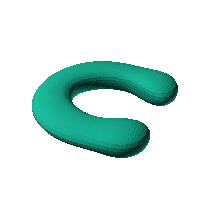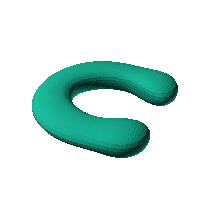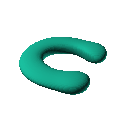Blob Farm# blob28The blob in all its glory:C shape, maybe a good case.

 Click on the snapshot to download the blob's stl file.Octave Code:
```  # name of the blob
project = "blob28";

function w = f(x2,y2,z2,c,r,e)
x  = (x2-c(1))/r(1);
y  = (y2-c(2))/r(2);
z  = (z2-c(3))/r(3);
# function at origin must be <0, and >0 far enough away.  w=0 defines the surface
k = -0.5;
j = -15;
theta = atan2(x,y);

w = ((0.3*x).^2 + (0.3*y + 0.0*(z).*(z - 1)).^2 + (z.^2));
w = w + 2./((0.8*x - 0.5).^2 + (y - 0.0).^2 + (z - 0).^2);
w = w - (0.3)./((x - 2).^2 + (y - 2).^2 + 8*z.^4);
w = w - (0.3)./((x - 2).^2 + (y + 2).^2 + 8*z.^4);
w = w-1;

endfunction;

c_outer = [0,0,0];
r_outer = [30,30,30];

step = 4;  # grid pitch in mm  start with 4mm to see the shape quickly.  Once you have it just right, change to 2mm for printing

source("../octave/func2stl_v01.m");  # do all the calculations
```
GNU Octave